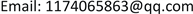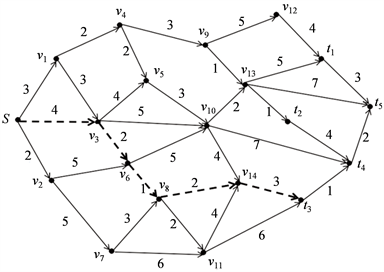﻿ 网络上指定起点与终点集的路径规划问题 A Path Planning Problem for Specified Starting Point and Ending Point Set on the Network

Vol. 08  No. 01 ( 2019 ), Article ID: 28353 , 6 pages
10.12677/AAM.2019.81001

A Path Planning Problem for Specified Starting Point and Ending Point Set on the Network

Simin Suo, Wei Li, Hua Yang

School of Mathematics and Computer Science, Yunnan Minzu University, Kunming YunnanReceived: Dec. 3rd, 2018; accepted: Dec. 31st, 2018; published: Jan. 7th, 2019ABSTRACT

Path planning has been applied in many fields, especially in logistics management. This paper takes logistics distribution in the process of online shopping as the background, the aim is to find a shortest path in the process of distribution. Considering the case of shipping from a warehouse to one of the districts with multiple distribution centers, such a problem is considered as the path planning problem of the specified starting point and ending point set on the network. And using Dijkstra algorithm to design a optimal algorithm to solve such a problem, it provides a new way to solve such problems.

Keywords:Path Planning, Shortest Path, Dijkstra Algorithm

㱔思敏，李伟，杨花1. 引言

2. 问题描述

Dijkstra算法具体过程如下：

Input：一个有赋权向图 $G=\left(V,E;c\right)$，权 $c:E\to {R}^{+}$，以及一个顶点 $s\in V$

Output：从s到图中所有顶点 $v\in V$ 的最短路及其长度

Begin

Step1 标记所有未被访问的节点，建立一个所有未被访问的节点的集合，叫做未被访问节点集，记做Q；

Step2 给每个节点分配一个暂定的距离值:初始节点设为 $l\left(s\right):=0$，对所有 $v\in V\\left\{s\right\}$，令 $l\left(v\right):=\infty$，令 $R:=\varnothing$ 是已访问的节点集合；

Step3 对于当前节点 $v\in V\\left\{s\right\}$，考虑它所有未访问的邻近的顶点，并计算它们通过当前节点的暂定距离。将新计算的暂定距离与当前分配的值进行比较，并修改当前标号为较小的值，使得 $l\left(v\right)=\underset{w\in V\R}{\mathrm{min}}l\left(w\right)$

Step4 当我们考虑到当前节点的所有未访问邻点时，将当前节点标记为已访问，并将其从未访问集合中删除，也即令 $R:=R\cup \left\{v\right\}$

Step5 对所有使得 $\left(v,w\right)\in E$$w\in V\R$，执行

$l\left(w\right)>l\left(v\right)+c\left(\left(v,w\right)\right)$，则

$l\left(w\right):=l\left(v\right)+c\left(\left(v,w\right)\right)$，且 $p\left(w\right):=v$

Step6 若 $R\ne V$，则转向Step3。

End

3. 算法设计与分析

Input: 一个赋权有向图 $G=\left(V,E;c\right)$，权 $c:E\to {R}^{+}$，以及一个顶点 $s\in V$，一个顶点集 $T\in V$，且 $s\notin T$

Output: 从s到T中所有顶点的最短路中最短的一条及其长度

Begin

Step1：置起点 $S:=\left\{s\right\}$，终点集 $T:=\left\{{v}_{1},{v}_{2},\cdot \cdot \cdot ,{v}_{|T|}\right\}$

Step2：利用算法1找出从起点s到 ${v}_{1}$ 的一条最短 $s-{v}_{1}$ 路；

Step3：再分别找出从起点s到 ${v}_{2},{v}_{3},\cdots ,{v}_{|T|-1}$ 的最短 $s-{v}_{2},s-{v}_{3},\cdots ,s-{v}_{|T|-1}$ 路；

Step4：最后找出从起点s到 ${v}_{|T|}$ 的最短 $s-{v}_{|T|}$

Step5：比较这 $|T|$ 条最短路的长度，输出其中最短的一条。

End

4. 应用举例Figure 1. A regional logistics distribution network

$l\left({v}_{1}\right)=c\left(s,{v}_{1}\right)=3$

$l\left({v}_{2}\right)=c\left(s,{v}_{2}\right)=2$

$l\left({v}_{3}\right)=c\left(s,{v}_{3}\right)=4$

$l\left({v}_{4}\right)=l\left({v}_{1}\right)+c\left({v}_{1}+{v}_{4}\right)=3+2=5$

$l\left({v}_{5}\right)=l\left({v}_{4}\right)+c\left({v}_{4},{v}_{5}\right)=5+2=7$

$l\left({v}_{6}\right)=l\left({v}_{3}\right)+c\left({v}_{3},{v}_{6}\right)=4+2=6$

$l\left({v}_{7}\right)=l\left({v}_{2}\right)+c\left({v}_{2},{v}_{7}\right)=2+5=7$

$l\left({v}_{8}\right)=l\left({v}_{6}\right)+c\left({v}_{6},{v}_{8}\right)=6+1=7$

$l\left({v}_{9}\right)=l\left({v}_{4}\right)+c\left({v}_{4},{v}_{9}\right)=5+3=8$

$l\left({v}_{10}\right)=l\left({v}_{2}\right)+c\left({v}_{2},{v}_{10}\right)=4+5=9$

$l\left({v}_{11}\right)=l\left({v}_{8}\right)+c\left({v}_{8},{v}_{11}\right)=7+2=9$

$l\left({v}_{12}\right)=l\left({v}_{9}\right)+c\left({v}_{9},{v}_{12}\right)=8+5=13$

$l\left({v}_{13}\right)=l\left({v}_{9}\right)+c\left({v}_{9},{v}_{13}\right)=8+\text{3}=\text{11}$

$l\left({v}_{14}\right)=l\left({v}_{8}\right)+c\left({v}_{8},{v}_{14}\right)=7+2=9$

$l\left({t}_{1}\right)=l\left({v}_{12}\right)+c\left({v}_{12},{t}_{1}\right)=13+4=17$

$l\left({t}_{2}\right)=l\left({v}_{13}\right)+c\left({v}_{13},{t}_{2}\right)=\text{11}+\text{2}=1\text{3}$

$l\left({t}_{3}\right)=l\left({v}_{14}\right)+c\left({v}_{14},{v}_{3}\right)=9+3=12$

$l\left({t}_{4}\right)=l\left({t}_{3}\right)+c\left({t}_{3},{t}_{4}\right)=12+1=13$

$l\left({t}_{5}\right)=l\left({t}_{5}\right)+c\left({t}_{4},{t}_{5}\right)=13+2=15$Figure 2. A shortest distribution path

5. 结论

㱔思敏,李 伟,杨 花. 网络上指定起点与终点集的路径规划问题
A Path Planning Problem for Specified Starting Point and Ending Point Set on the Network[J]. 应用数学进展, 2019, 08(01): 1-6. https://doi.org/10.12677/AAM.2019.81001

1. 1. 丛岩峰. 基于滚动优化原理的路径规划方法研究[D]: [硕士学位论文]. 长春: 吉林大学, 2007.

2. 2. Latombe, J.C. (2012) Robot Motion Planning. Springer Science & Business Media.

3. 3. Dijkstra, E.W. (1959) A Note on Two Problems in Connexion with Graphs. Numerische Mathematik, 1, 269-271. https://doi.org/10.1007/BF01386390

4. 4. 余震江. 基于最短路径Dijkstra算法的铁路客运中转径路优化研究[D]: [硕士学位论文]. 重庆: 重庆大学, 2008.

5. 5. 韩慧玲, 胡红萍. Dijkstra算法在公交换乘最短路径中的应用[J]. 硅谷, 2011(21): 111+126.

6. 6. Kirk, J. Dijkstra’s Minimum Cost Path Algorithm. https://cn.mathworks.com/matlabcentral/fileexchange/20025-dijkstra-s-minimum-cost-path-algorithm

7. 7. 李健. 基于Dijkstra最短路径算法的优化研究[J]. 渭南师范学院学报, 2009, 24(5): 61-64.